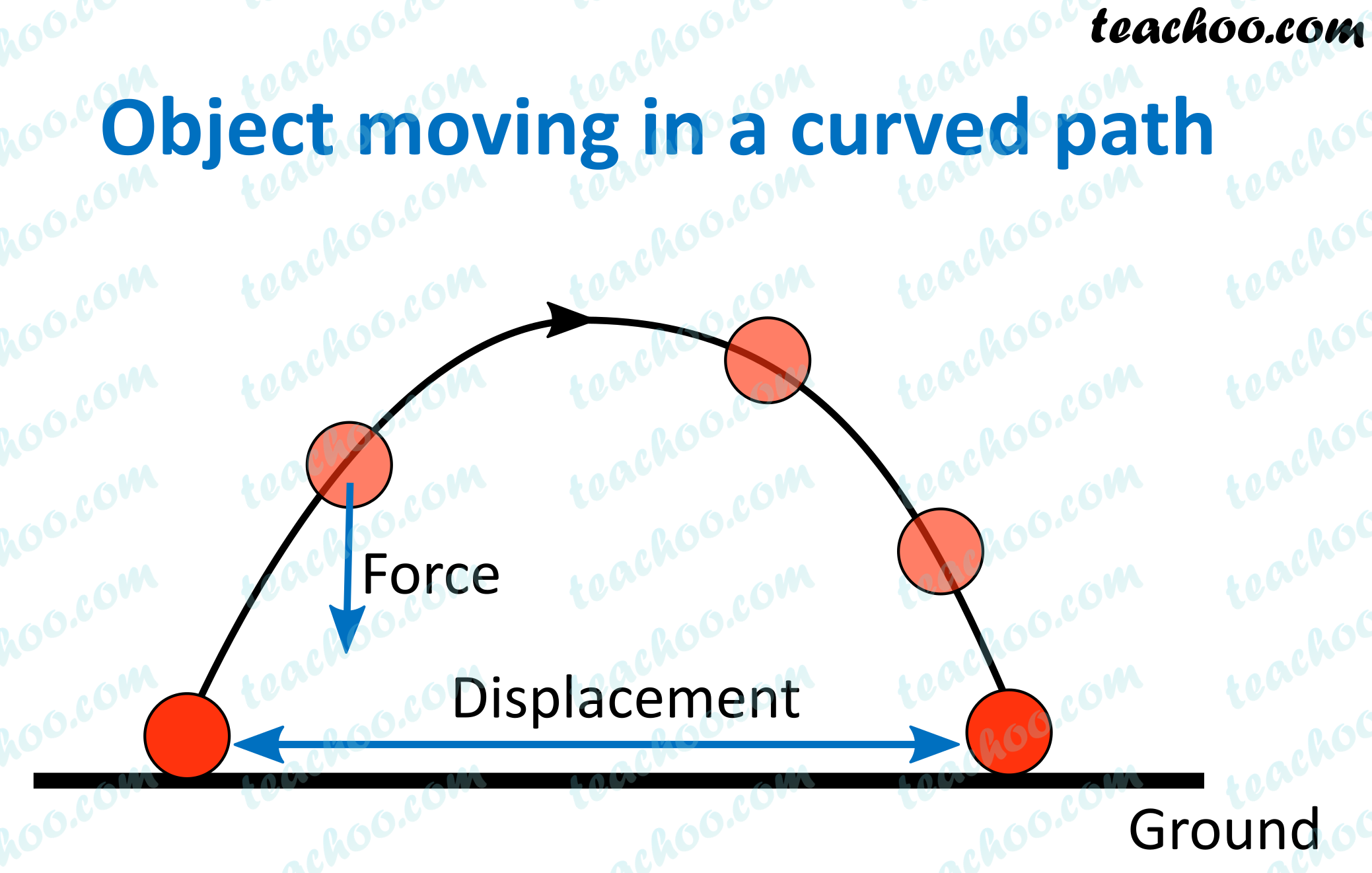NCERT Questions

Class 9
Chapter 11 Class 9 - Work and Energy (Term 2)

## An object thrown at a certain angle to the ground moves in a curved path and falls back to the ground. The initial and the final points of the path of the object lie on the same horizontal line. What is the work done by the force of gravity on the object?

###(adsbygoogle = window.adsbygoogle || []).push({});

Work is done when force applied produces motion in the direction of the force.

In this case,

Force is applied in the vertical direction

But the displacement of the body is in the horizontal direction.

Since angle between force and displacement is 90 degrees.

Hence, no work is done